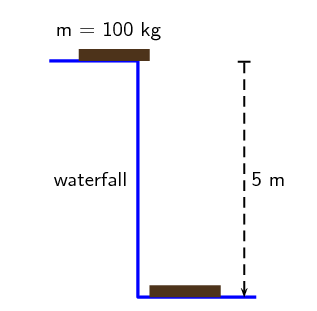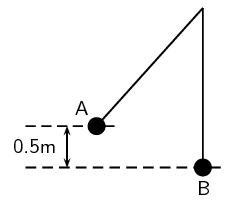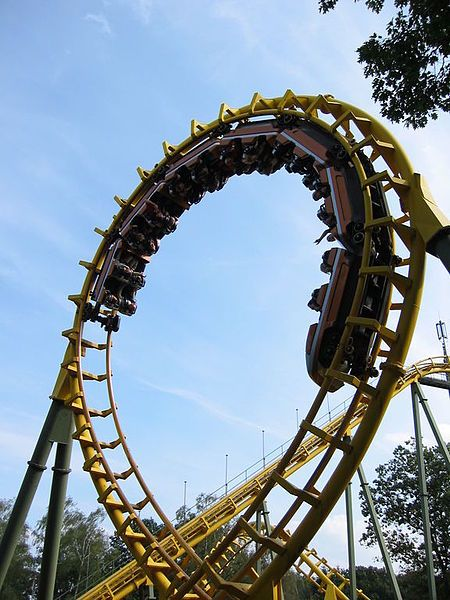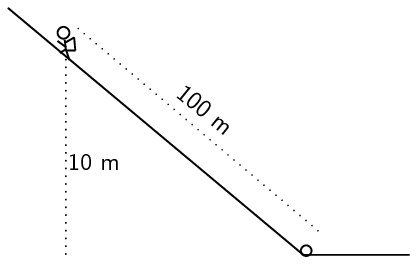We think you are located in United States. Is this correct?

# 22.5 Conservation of mechanical energy

## 22.5 Conservation of mechanical energy (ESAHO)

Conservation of Energy

The Law of Conservation of Energy: Energy cannot be created or destroyed, but is merely changed from one form into another.

So far we have looked at two types of energy: gravitational potential energy and kinetic energy. The sum of the gravitational potential energy and kinetic energy is called the mechanical energy. In a closed system, one where there are no external dissipative forces acting, the mechanical energy will remain constant. In other words, it will not change (become more or less). This is called the Law of Conservation of Mechanical Energy.

In problems involving the use of conservation of energy, the path taken by the object can be ignored. The only important quantities are the object's velocity (which gives its kinetic energy) and height above the reference point (which gives its gravitational potential energy).

Conservation of mechanical energy

Law of Conservation of Mechanical Energy: The total amount of mechanical energy, in a closed system in the absence of dissipative forces (e.g. friction, air resistance), remains constant.

This means that potential energy can become kinetic energy, or vice versa, but energy cannot “disappear”. For example, in the absence of air resistance, the mechanical energy of an object moving through the air in the Earth's gravitational field, remains constant (is conserved).

### Using the law of conservation of energy (ESAHP)

Mechanical energy is conserved (in the absence of friction). Therefore we can say that the sum of the $${E}_{P}$$ and the $${E}_{K}$$ anywhere during the motion must be equal to the sum of the the $${E}_{P}$$ and the $${E}_{K}$$ anywhere else in the motion.

We can now apply this to the example of the suitcase on the cupboard. Consider the mechanical energy of the suitcase at the top and at the bottom. We can say:

\begin{align*} {E}_{M1} & = {E}_{M2} \\ {E}_{P1} + {E}_{K1} & = {E}_{P2} + {E}_{K2} \\ mgh + \frac{1}{2}m{v}^{2} & = mgh + \frac{1}{2}m{v}^{2} \\ \left(\text{1}\text{ kg}\right)\left(\text{9,8}\text{ m·s$^{-2}$}\right)\left(\text{2}\text{ m}\right) + 0 & = 0 + \frac{1}{2}\left(\text{1}\text{ kg}\right)\left({v}^{2}\right) \\ \text{19,6} & = \frac{1}{2}\left({v}^{2}\right) \\ {v}^{2} & = \text{39,2}\text{ m$^{2}$·s$^{-2}$} \\ v & = \text{6,26}\text{ m·s$^{-1}$} \end{align*}

The suitcase will strike the ground with a velocity of $$\text{6,26}$$ $$\text{m·s^{-1}}$$.

From this we see that when an object is lifted, like the suitcase in our example, it gains potential energy. As it falls back to the ground, it will lose this potential energy, but gain kinetic energy. We know that energy cannot be created or destroyed, but only changed from one form into another. In our example, the potential energy that the suitcase loses is changed to kinetic energy.

The suitcase will have maximum potential energy at the top of the cupboard and maximum kinetic energy at the bottom of the cupboard. Halfway down it will have half kinetic energy and half potential energy. As it moves down, the potential energy will be converted (changed) into kinetic energy until all the potential energy is gone and only kinetic energy is left. The $$\text{19,6}$$ $$\text{J}$$ of potential energy at the top will become $$\text{19,6}$$ $$\text{J}$$ of kinetic energy at the bottom.

## Conversion of energy

### Materials

A length of plastic pipe with diameter approximately 20 mm, a marble, some masking tape and a measuring tape.

### To do (1)

First put one end of the pipe on the table top so that it is parallel to the top of the table and tape it in position with the masking tape.

Lift the other end of the pipe upwards and hold it at a steady height not too high above the table.

Measure the vertical height from the table top to the top opening of the pipe.

Now put the marble at the top of the pipe and let it go so that it travels through the pipe and out the other end.

### Questions

• What is the velocity (i.e. fast, slow, not moving) of the marble when you first put it into the top of the pipe and what does this mean for its gravitational potential and kinetic energy?

• What is the velocity (i.e. fast, slow, not moving) of the marble when it reaches the other end of the pipe and rolls onto the desk? What does this mean for its gravitational potential and kinetic energy?

### To do (2)

Now lift the top of the pipe as high as it will go.

Measure the vertical height of the top of the pipe above the table top.

Put the marble into the top opening and let it roll through the pipe onto the table.

### Questions

• What is the velocity (i.e. fast, slow, not moving) of the marble when you put it into the top of the pipe, and what does this mean for its gravitational potential and kinetic energy?

• Compared to the first attempt, what was different about the height of the top of the tube? How do you think this affects the gravitational potential energy of the marble?

• Compared to your first attempt, was the marble moving faster or slower when it came out of the bottom of the pipe the second time? What does this mean for the kinetic energy of the marble?

The activity with the marble rolling down the pipe shows very nicely the conversion between gravitational potential energy and kinetic energy. In the first instance, the pipe was held relatively low and therefore the gravitational potential energy was also relatively low. The kinetic energy at this point was zero since the marble wasn't moving yet. When the marble rolled out of the other end of the pipe, it was moving relatively slowly, and therefore its kinetic energy was also relatively low. At this point its gravitational potential energy was zero since it was at zero height above the table top.

In the second instance, the marble started off higher up and therefore its gravitational potential energy was higher. By the time it got to the bottom of the pipe, its gravitational potential energy was zero (zero height above the table) but its kinetic energy was high since it was moving much faster than the first time. Therefore, the gravitational potential energy was converted completely to kinetic energy (if we ignore friction with the pipe).

In the case of the pipe being held higher, the gravitational potential energy at the start was higher, and the kinetic energy (and velocity) of the marble was higher at the end. In other words, the total mechanical energy was higher and and only depended on the height you held the pipe above the table top and not on the distance the marble had to travel through the pipe.

## Worked example 7: Using the Law of Conservation of Mechanical Energy

During a flood a tree trunk of mass $$\text{100}$$ $$\text{kg}$$ falls down a waterfall. The waterfall is $$\text{5}$$ $$\text{m}$$ high.If air resistance is ignored, calculate:

• the potential energy of the tree trunk at the top of the waterfall.

• the kinetic energy of the tree trunk at the bottom of the waterfall.

• the magnitude of the velocity of the tree trunk at the bottom of the waterfall.

### Analyse the question to determine what information is provided

• The mass of the tree trunk $$m = \text{100}\text{ kg}$$

• The height of the waterfall $$h = \text{5}\text{ m}$$.

These are all in SI units so we do not have to convert.

### Analyse the question to determine what is being asked

• Potential energy at the top

• Kinetic energy at the bottom

• Velocity at the bottom

### Calculate the potential energy at the top of the waterfall.

\begin{align*} {E}_{P} & = mgh \\ & = \left(\text{100}\text{ kg}\right)\left(\text{9,8}\text{ m·s$^{-2}$}\right)\left(\text{5}\text{ m}\right) \\ & = \text{4 900}\text{ J} \end{align*}

### Calculate the kinetic energy at the bottom of the waterfall.

The total mechanical energy must be conserved.

${E}_{K1} + {E}_{P1} = {E}_{K2} + {E}_{P2}$

Since the trunk's velocity is zero at the top of the waterfall, $${E}_{K1}=0$$.

At the bottom of the waterfall, $$h = \text{0}\text{ m}$$, so $${E}_{P2}=0$$.

Therefore $${E}_{P1} = {E}_{K2}$$ or in words:

The kinetic energy of the tree trunk at the bottom of the waterfall is equal to the potential energy it had at the top of the waterfall. Therefore $${E}_{K} = \text{4 900}\text{ J}$$

### Calculate the velocity at the bottom of the waterfall.

To calculate the velocity of the tree trunk we need to use the equation for kinetic energy.

\begin{align*} {E}_{K} & = \frac{1}{2}m{v}^{2} \\ \text{4 900} & = \frac{1}{2}\left(\text{100}\text{ kg}\right)\left({v}^{2}\right) \\ 98 & = {v}^{2} \\ v & = \text{9,899...}\text{ m·s$^{-1}$} \\ v & = \text{9,90}\text{ m·s$^{-1}$} \end{align*}

## Worked example 8: Pendulum

A $$\text{2}$$ $$\text{kg}$$ metal ball is suspended from a rope as a pendulum. If it is released from point A and swings down to the point B (the bottom of its arc):

1. show that the velocity of the ball is independent of its mass,

2. calculate the velocity of the ball at point B.### Analyse the question to determine what information is provided

• The mass of the metal ball is $$m = \text{2}\text{ kg}$$

• The change in height going from point A to point B is $$h = \text{0,5}\text{ m}$$

• The ball is released from point A so the velocity at point, $${v}_{A} = \text{0}\text{ m·s^{-1}}$$.

All quantities are in SI units.

### Analyse the question to determine what is being asked

• Prove that the velocity is independent of mass.

• Find the velocity of the metal ball at point B.

### Apply the Law of Conservation of Mechanical Energy to the situation

Since there is no friction, mechanical energy is conserved. Therefore:

\begin{align*} {E}_{M1} & = {E}_{M2} \\ {E}_{P1} + {E}_{K1} & = {E}_{P2} + {E}_{K2} \\ mg{h}_{1} + \frac{1}{2}m{\left({v}_{1}\right)}^{2} & = mg{h}_{2} + \frac{1}{2}m{\left({v}_{2}\right)}^{2} \\ mg{h}_{1} + 0 & = 0 + \frac{1}{2}m{\left({v}_{2}\right)}^{2} \\ mg{h}_{1} & = \frac{1}{2}m{\left({v}_{2}\right)}^{2} \end{align*}

The mass of the ball $$m$$ appears on both sides of the equation so it can be eliminated so that the equation becomes:

\begin{align*} g{h}_{1} & = \frac{1}{2}{\left({v}_{2}\right)}^{2} \\ 2g{h}_{1} & = {\left({v}_{2}\right)}^{2} \end{align*}

This proves that the velocity of the ball is independent of its mass. It does not matter what its mass is, it will always have the same velocity when it falls through this height.

### Calculate the velocity of the ball at point B

We can use the equation above, or do the calculation from “first principles”:

\begin{align*} {\left({v}_{2}\right)}^{2} & = 2g{h}_{1} \\ {\left({v}_{2}\right)}^{2} & = \left(2\right)\left(\text{9,8}\text{ m·s$^{-2}$}\right)\left(\text{0,5}\text{ m}\right) \\ {\left({v}_{2}\right)}^{2} & = \text{9,8}\text{ m$^{2}$·s$^{-2}$} \\ {v}_{2} & = \sqrt{\text{9,8}\text{ m$^{2}$·s$^{-2}$}} \\ {v}_{2} & = \text{3,13}\text{ m·s$^{-1}$} \end{align*}

Alternatively you can do:

\begin{align*} {E}_{K1} + {E}_{P1} & = {E}_{K2} + {E}_{P2} \\ mg{h}_{1} + \frac{1}{2}m{\left({v}_{1}\right)}^{2} & = mg{h}_{2} + \frac{1}{2}m{\left({v}_{2}\right)}^{2} \\ mg{h}_{1} + 0 & = 0 + \frac{1}{2}m{\left({v}_{2}\right)}^{2} \\ {\left({v}_{2}\right)}^{2} & = \frac{2mg{h}_{1}}{m} \\ {\left({v}_{2}\right)}^{2} & = \frac{2\left(\text{2}\text{ kg}\right)\left(\text{9,8}\text{ m·s$^{-2}$}\right)\left(\text{0,5}\text{ m}\right)}{\text{2}\text{ kg}} \\ {v}_{2} & = \sqrt{\text{9,8}\text{ m$^{2}$·s$^{-2}$}} \\ {v}_{2} & = \text{3,13}\text{ m·s$^{-1}$} \end{align*}

## Worked example 9: The roller coaster

A roller coaster ride at an amusement park starts from rest at a height of $$\text{50}$$ $$\text{m}$$ above the ground and rapidly drops down along its track. At some point, the track does a full 360° loop which has a height of $$\text{20}$$ $$\text{m}$$, before finishing off at ground level. The roller coaster train itself with a full load of people on it has a mass of $$\text{850}$$ $$\text{kg}$$.

Roller coasterIf the roller coaster and its track are frictionless, calculate:

1. the velocity of the roller coaster when it reaches the top of the loop

2. the velocity of the roller coaster at the bottom of the loop (i.e. ground level)

### Analyse the question to determine what information is provided

• The mass of the roller coaster is $$m = \text{850}\text{ kg}$$

• The initial height of the roller coaster at its starting position is $${h}_{1} = \text{50}\text{ m}$$

• The roller coaster starts from rest, so its initial velocity $${v}_{1} = \text{0}\text{ m·s^{-1}}$$

• The height of the loop is $${h}_{2} = \text{20}\text{ m}$$

• The height at the bottom of the loop is at ground level, $${h}_{3} = \text{0}\text{ m}$$

We do not need to convert units as they are in the correct form already.

### Analyse the question to determine what is being asked

• the velocity of the roller coaster at the top of the loop

• the velocity of the roller coaster at the bottom of the loop

### Calculate the velocity at the top of the loop

From the conservation of mechanical energy, We know that at any two points in the system, the total mechanical energy must be the same. Let's compare the situation at the start of the roller coaster to the situation at the top of the loop:

\begin{align*} {E}_{M1} & = {E}_{M2} \\ {E}_{K1} + {E}_{P1} & = {E}_{K2} + {E}_{P2} \\ 0 + mg{h}_{1} & = \frac{1}{2}m{\left({v}_{2}\right)}^{2} + mg{h}_{2} \end{align*}

We can eliminate the mass, $$m$$, from the equation by dividing both sides by $$m$$.

\begin{align*} g{h}_{1} & = \frac{1}{2}{\left({v}_{2}\right)}^{2} + g{h}_{2} \\ {\left({v}_{2}\right)}^{2} & = 2\left(g{h}_{1} - g{h}_{2}\right) \\ {\left({v}_{2}\right)}^{2} & = 2\left(\left(\text{9,8}\text{ m·s$^{-2}$}\right)\left(\text{50}\text{ m}\right)-\left(\text{9,8}\text{ m·s$^{-2}$}\right)\left(\text{20}\text{ m}\right)\right) \\ {v}_{2} & = \text{24,25}\text{ m·s$^{-1}$} \end{align*}

### Calculate the velocity at the bottom of the loop

Again we can use the conservation of energy and the total mechanical energy at the bottom of the loop should be the same as the total mechanical energy of the system at any other position. Let's compare the situations at the start of the roller coaster's trip and the bottom of the loop:

\begin{align*} {E}_{M1} & = {E}_{M3} \\ {E}_{K1} + {E}_{P1} & = {E}_{K3} + {E}_{P3} \\ \frac{1}{2}{m}_{1}{\left(0\right)}^{2} + mg{h}_{1} & = \frac{1}{2}m{\left({v}_{3}\right)}^{2} + mg\left(0\right) \\ mg{h}_{1} & = \frac{1}{2}m{\left({v}_{3}\right)}^{2} \\ {\left({v}_{3}\right)}^{2} & = 2g{h}_{1} \\ {\left({v}_{3}\right)}^{2} & = 2\left(\text{9,8}\text{ m·s$^{-2}$}\right)\left(\text{50}\text{ m}\right) \\ {v}_{3} & = \text{31,30}\text{ m·s$^{-1}$} \end{align*}

## Worked example 10: An inclined plane

A mountain climber who is climbing a mountain in the Drakensberg during winter, by mistake drops her water bottle which then slides $$\text{100}$$ $$\text{m}$$ down the side of a steep icy slope to a point which is 10 m lower than the climber's position. The mass of the climber is $$\text{60}$$ $$\text{kg}$$ and her water bottle has a mass of $$\text{500}$$ $$\text{g}$$ .

1. If the bottle starts from rest, how fast is it travelling by the time it reaches the bottom of the slope? (Neglect friction.)

2. What is the total change in the climber's potential energy as she climbs down the mountain to fetch her fallen water bottle? i.e. what is the difference between her potential energy at the top of the slope and the bottom of the slope?### Analyse the question to determine what information is provided

• the distance travelled by the water bottle down the slope, $$d = \text{100}\text{ m}$$

• the difference in height between the starting position and the final position of the water bottle is $$h = \text{10}\text{ m}$$

• the bottle starts sliding from rest, so its initial velocity is $${v}_{1} = \text{9,8}\text{ m·s^{-1}}$$

• the mass of the climber is $$\text{60}$$ $$\text{kg}$$

• the mass of the water bottle is $$\text{500}$$ $$\text{g}$$. We need to convert this mass into $$\text{kg}$$: $$\text{500}\text{ g} = \text{0,5}\text{ kg}$$

### Analyse the question to determine what is being asked

• What is the velocity of the water bottle at the bottom of the slope?

• What is the difference between the climber's potential energy when she is at the top of the slope compared to when she reaches the bottom?

### Calculate the velocity of the water bottle when it reaches the bottom of the slope

\begin{align*} {E}_{M1} & = {E}_{M2} \\ {E}_{K1} + {E}_{P1} & = {E}_{K2} + {E}_{P2} \\ \frac{1}{2}m{\left({v}_{1}\right)}^{2} + mg{h}_{1} & = \frac{1}{2}m{\left({v}_{2}\right)}^{2} + mg{h}_{2} \\ 0 + mg{h}_{1} & = \frac{1}{2}m{\left({v}_{2}\right)}^{2} + 0 \\ {\left({v}_{2}\right)}^{2} & = \frac{2mgh}{m} \\ {\left({v}_{2}\right)}^{2} & = 2gh \\ {\left({v}_{2}\right)}^{2} & = \left(2\right)\left(\text{9,8}\text{ m·s$^{-2}$}\right)\left(\text{10}\text{ m}\right) \\ {v}_{2} & = \text{14}\text{ m·s$^{-1}$} \end{align*}

Note: the distance that the bottle travelled (i.e. $$\text{100}$$ $$\text{m}$$) does not play any role in calculating the energies. It is only the height difference that is important in calculating potential energy.

### Calculate the difference between the climber's potential energy at the top of the slope and her potential energy at the bottom of the slope

At the top of the slope, her potential energy is:

\begin{align*} {E}_{P1} & = mg{h}_{1} \\ & = \left(\text{60}\text{ kg}\right)\left(\text{9,8}\text{ m·s$^{-2}$}\right)\left(\text{10}\text{ m}\right) \\ & = \text{5 800}\text{ J} \end{align*}

At the bottom of the slope, her potential energy is:

\begin{align*} {E}_{P1} & = mg{h}_{1} \\ & = \left(\text{60}\text{ kg}\right)\left(\text{9,8}\text{ m·s$^{-2}$}\right)\left(\text{0}\text{ m}\right) \\ & = \text{0}\text{ J} \end{align*}

Therefore the difference in her potential energy when moving from the top of the slope to the bottom is:

${E}_{P1} - {E}_{P2} = \text{5 880} - 0 = \text{5 880}\text{ J}$

## Potential energy

Textbook Exercise 22.3

A tennis ball, of mass $$\text{120}$$ $$\text{kg}$$ , is dropped from a height of $$\text{5}$$ $$\text{m}$$ . Ignore air friction.

1. What is the potential energy of the ball when it has fallen $$\text{3}$$ $$\text{m}$$ ?

2. What is the velocity of the ball when it hits the ground?

Solution not yet available

A ball rolls down a hill which has a vertical height of $$\text{15}$$ $$\text{m}$$ . Ignoring friction, what would be the

1. gravitational potential energy of the ball when it is at the top of the hill?

2. velocity of the ball when it reaches the bottom of the hill?

Solution not yet available

A bullet, mass $$\text{50}$$ $$\text{g}$$ , is shot vertically up in the air with a muzzle velocity of $$\text{200}$$ $$\text{m·s^{-1}}$$. Use the Principle of Conservation of Mechanical Energy to determine the height that the bullet will reach. Ignore air friction.

Solution not yet available

A skier, mass $$\text{50}$$ $$\text{kg}$$, is at the top of a $$\text{6,4}$$ $$\text{m}$$ ski slope.

1. Determine the maximum velocity that she can reach when she skis to the bottom of the slope.

2. Do you think that she will reach this velocity? Why/Why not?

Solution not yet available

A pendulum bob of mass $$\text{1,5}$$ $$\text{kg}$$, swings from a height A to the bottom of its arc at B. The velocity of the bob at B is $$\text{4}$$ $$\text{m·s^{-1}}$$. Calculate the height A from which the bob was released. Ignore the effects of air friction.

Solution not yet available

Prove that the velocity of an object, in free fall, in a closed system, is independent of its mass.

Solution not yet available